Home

ARTICLE 7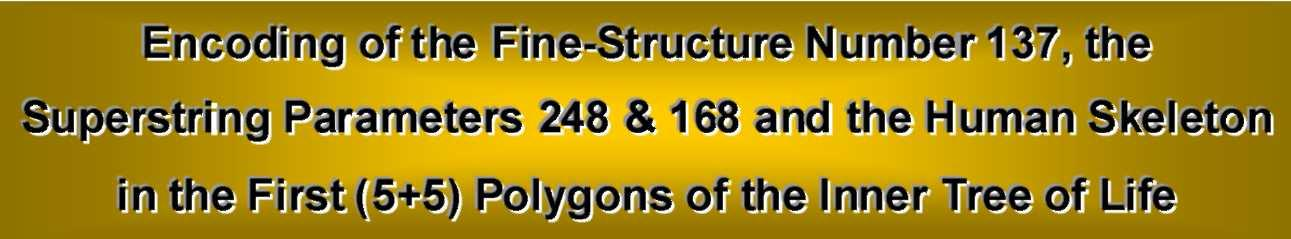by

Stephen M. Phillips

Flat 4, Oakwood House, 117-119 West Hill Road. Bournemouth. Dorset BH2 5PH. England.

Website: http://smphillips.mysite.com

1. Patterns within patterns
In Article 4  it was shown how the ten Hebrew Divine Names mathematically prescribe different subsets of what the author has discovered  to be the inner form of the Kabbalistic Tree of Life. Generated in posse by the geometry of its outer shape, this hidden structure consists of two similar sets of seven regular polygons: triangle, square, pentagon, hexagon, octagon, decagon and dodecagon (Fig. 1). They are enfolded in one another, sharing a so-called ‘root edge,’ from which each polygon is generated in turn on either side of it as mirror images of each other. Although this geometrical construction has long been known in a formal sense to mathematicians, they have never understood its true significance, which transcends issues of pure geometry, requiring the cipher of the Pythagorean tetractys and an understanding of the Divine Names and their gematria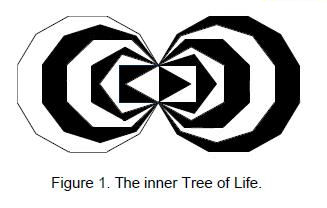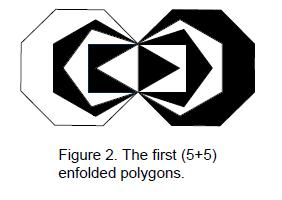numbers to decode the information about the nature of spiritual and physical reality that this cosmic blueprint contains. In Article 4 — and in more detail in the author’s book — a subset of the fourteen regular polygons consisting of the seven polygons enfolded on one side of the root edge and the five polygons with most corners on its other side was shown to encode the Tree of Life mapping of what Theosophists call the ‘seven cosmic planes of consciousness — what in my book and in articles on this website I have called the ‘Cosmic Tree of Life’ (CTOL). The first six regular polygons on each side of their shared root edge have been shown  to encode the number 1680 as the structural parameter of the E8×E8 heterotic superstring constituents of up and down quarks (seeprevious articles). Both these subsets of the complete set of polygons were demonstrated to be ‘Tree of Life patterns’ as well because their properties are prescribed by the gematria numbers of the ten Hebrew Godnames. As Article 3 stated , this is a necessary condition for an object to constitute what writers all too loosely call ‘sacred geometry.’ This article will explore the properties of a new subset, namely, the first five of the seven polygons (Fig. 2) enfolded on either side of their shared root edge. Their prescription by the Divine Names indicates that these (5+5) regular polygons, too, constitute a Tree of Life pattern. It indicates that they should embody basic parameters of the superstring encountered inprevious discussions of such patterns. The article will confirm this expectation in a remarkable way.

2. Properties of the first five regular polygons
The basic properties of the five regular polygons shown in Figure 2 are listed below. The case of separate polygons is examined first and then the enfolded polygons are analysed. Both sets of polygons are also considered in each case. Numbers in boldface will indicate number values of Sephiroth, their Godnames, Archangelic Names, Angelic Names & Mundane Chakras (see Table 1). Each polygon is divided into its

1

Table 1. Number values of the Sephiroth.
(All such numbers appearing in text are written in
boldface. Cited numbers are in coloured boxes)

 Sephirah Title Godname Archangel Order of Angels Mundane Chakra Kether 620 21 314 833 636 Chokmah 73 15, 26 248 187 140 Binah 67 50 311 282 317 Chesed 72 31 62 428 194 Geburah 216 36 131 630 95 Tiphareth 1081 76 101 140 640 Netzach 148 129 97 1260 64 Hod 15 153 311 112 48 Yesod 80 49 246 272 87 Malkuth 496 65, 155 280 351 168

triangular sectors, which are then turned into tetractyses. ‘Hexagonal yods’ (so-called because they form the corners and centre of a hexagon) are those yods that are not corners of tetractyses.

 triangle square pentagon hexagon octagon Number of corners = 3 4 5 6 8 Number of yods = 19 25 31 37 49

5 separate polygons

1. Number of corners of polygons = 3 + 4 + 5 + 6 + 8 = 26;

2. Number of sides of polygons = 26;

3. Number of corners and sides of polygons = 26 + 26 = 52;

4. Number of sectors = 26;

5. Number of corners of 26 sectors = 26 + 5 = 31;

6. Number of sides of sectors = 2×26 = 52;

7. Number of corners and sides of sectors = 31 + 52 = 83;

8. Number of sides and sectors = 52 + 26 = 78;

9. Number of sectors and their corners and sides = 83 + 26 = 109;

10. Including their separate root edge, number of geometrical elements = 109 + 3 = 112;

11. Number of hexagonal yods = 5×26 =130;

12. Number of yods = 6×26 + 5 = 161;

13. Number of yods on boundaries of polygons = 26 + 2×26 = 78 (52 hexagonal);

14. Number of yods on boundaries of tetractyses = 26 + 2×26 + 2×26 + 5 = 135.

(5+5) separate polygons

1. Number of corners of polygons = 2×26 = 52;

2. Number of sides of polygons = 2×26 = 52;

3. Number of corners and sides of polygons = 2×52 = 104;

4. Number of sectors = 2×26 = 52;

5. Number of corners of sectors = 2×31 = 62;

6. Number of sides of sectors = 2×52 = 104;

7. Number of corners and sides of sectors = 62 + 104 = 166;

8. Number of sides and sectors = 104 + 52 = 156;

9. Number of sectors and their corners and sides = 166 + 52 = 218;

10. Including their root edge, number of geometrical elements = 218 + 3 = 221. These include (2+31+31=64) corners;

11. Number of hexagonal yods = 2×130 = 260 = 26×10;

12. Number of yods = 2×161 = 322;

13. Number of yods on boundaries of polygons = 2×78 = 156 (104 hexagonal);

14. Number of yods on boundaries of tetractyses = 2×135 = 270 (260 surround their centres).

5 enfolded polygons

1. Number of corners of polygons = 26 – 4×2 = 18 (16 outside root edge);

2. Number of sides of polygons = 26 – 4 = 22 (21 outside root edge);

2

1. Number of corners and sides of polygons = 18 + 22 = 40 (37 outside root edge);

2. Number of sectors = 25;

3. Number of corners of sectors = 31 – 4×2 – 1 = 22 (20 outside root edge);

4. Number of sides of sectors = 52 – 4 –2 = 46 (45 outside root edge);

5. Number of corners and sides of sectors = 22 + 46 = 68 (65 outside root edge);

6. Number of sides and sectors = 46 + 25 = 71 (70 outside root edge);

7. Number of sectors and their corners and sides = 68 + 25 = 93 (90 outside root edge);

8. Number of hexagonal yods = 130 – 4×2 – 2 – 2 – 1 = 117 (115 outside root edge);

9. Number of yods = 161 – 4×4 – 2 – 2 – 1 – 1 = 139 (135 outside root edge);

10. Number of yods on boundaries of polygons = 22×2 + 18 = 62 (58 outside root edge);

11. Number of yods on boundaries of tetractyses = 22 + 46×2 = 114 (110 outside root edge).

(5+5) enfolded polygons

1. Number of corners of polygons = 2×16 + 2 = 34 (32 outside root edge);

2. Number of sides of polygons = 2×21 + 1 = 43 (42 outside root edge);

3. Number of corners & sides of polygons = 34 + 43 = 77 (74 outside root edge, 73 intrinsic to polygons);

4. Number of sectors = 2×25 = 50;

5. Number of corners of sectors = 2×20 + 2 = 42 (40 outside root edge);

6. Number of sides of sectors = 2×45 + 1 = 91 (90 outside root edge);

7. Number of corners and sides of sectors = 2×65 + 3 = 133 (130 outside root edge);

8. Number of sides and sectors = 2×70 + 1 = 141 (140 outside root edge);

9. Number of sectors and their corners and sides = 2×90 + 3 = 183 (180 outside root edge);

10. Number of hexagonal yods = 2×115 + 2 = 232 (230 outside root edge);

11. Number of yods = 2×135 + 4 = 274 (270 outside root edge, 272 per set of 10 polygons);

12. Number of yods on boundaries of polygons = 2×58 + 4 = 120 (116 outside root edge). Number of yods inside polygons = 274 – 120 = 154 (77 per set of 5 polygons);

13. Number of yods on boundaries of tetractyses = 2×110 + 4 = 224 (220 outside root edge); Number of yods other than corners of polygons = 274 – 34 = 240. Of these, (4+4=8) are centres of polygons, leaving 232 yods that are not corners of tetractyses.

3. The holistic nature of the first five polygons
Set out below are ways in which properties of the sets of five and (5+5) polygons are prescribed by the number values of the Godnames of the ten Sephiroth:

 Kether: 21 21 sides of 5 enfolded polygons outside root edge; Chokmah: 15 93 tetractyses and their corners and sides in 5 enfolded polygons, where 93 = 47th odd integer and 47 = 15th prime number. Also, 5 enfolded polygons have 47 corners and tetractyses; 26 26 corners/sides/tetractyses in 5 separate polygons. Number of hexagonal yods in (5+5) separate polygons = 260 = 26×10. This is also the number of yods around their centres; Binah: 50 (5+5) enfolded polygons have 50 tetractyses; Chesed: 31 31 corners of 26 tetractyses in 5 separate polygons; Geburah: 36 Number of sides and tetractyses of 5 enfolded polygons = 71 = 36th odd integer; Tiphareth: 76 Number of yods inside boundaries of 5 enfolded polygons = 77 = 76th integer after 1. This is also the number of corners & sides of the (5+5) polygons; Netzach: 129 Number of hexagonal yods in 5 separate polygons = 130 = 129th integer after 1; Hod: 153 Number of yods inside (5+5) enfolded polygons = 154 = 153rd integer after 1; Yesod: 49 Number of tetractyses in (5+5) enfolded polygons = 50 = 49th integer after 1; Malkuth: 65 Number of hexagonal yods in 5 separate polygons = 130 = 65th even integer. Also, 65 corners & sides of tetractyses outside root edge of 5 enfolded polygons; 155 Number of tetractyses & their sides in 5 separate polygons = 156 = 155th integer after 1. Also, there are 156 yods on the boundaries of the (5+5) separate polygons.

General discussion

It is clear that the Godname numbers appear too naturally in the properties of the two sets of polygons for chance to play a role. That 27 (over 50%) of the 52 numbers shown in Table 1 should appear in the analysis of this article is too many to be coincidental. Indeed, further analysis would reveal other number values in the table. It is significant that the 50 sectors of the two sets of five enfolded polygons prescribed by ELOHIM, the Godname of Binah with number value 50, have 90 edges outside their root edge. This is because the number 90 is the sum of the ten numbers of Plato’s Lambda Tetractys discussed in Article 11 . It is shown in many articles published on this website to be a defining parameter of holistic systems (for example, the five Platonic solids with 50 vertices and 50 faces have 90 edges). The 20 sectors of the pair of

3

enfolded pentagons and hexagons (note that one sector of each hexagon is filled by a triangle) have 36 edges outside the root edge; the 30 sectors of the pairs of enfolded triangles, squares & octagons have 54 external edges. This 36:54 division reproduces the archetypal property of the Lambda Tetractys, wherein the sum of the numbers at its corners is 36 and the sum of the seven remaining numbers is 54:

 1 2    3 4    6    9 8  12   18   27 = 36 + 54

Moreover, according to (9) in the list of properties of the five enfolded polygons, 90 more geometrical elements are needed to construct them, starting from their shared base, of which 65 are corners & edges and 25 are triangles. This 65:25 division is reproduced in the Lambda Tetractys as, respectively, the sum of the four integers at its base and the sum of its six other integers: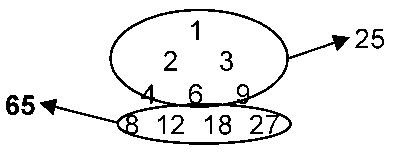Apart from their prescription by the Godnames, the first (5+5) enfolded polygons conform to a Tree of Life pattern because each set has 70 sectors & edges outside the root edge (Fig. 3). This compares with 1.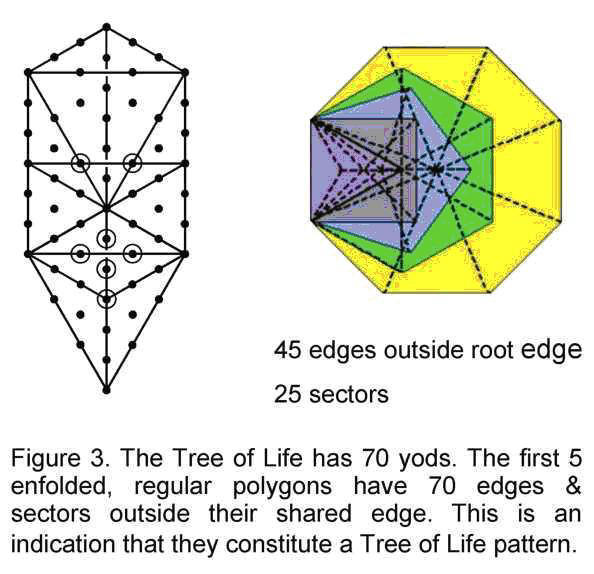the 70 yods in a Tree of Life whose 16 triangles are tetractyses, 2. the 70 corners of the (7+7) enfolded polygons, and 3. the 70 tetractyses of the first (6+6) enfolded polygons (Fig. 4), a set of polygons which was shown in Article 4 to be prescribed by the Godnames . Together with their prescription by the Divine Names, these properties are evidence for the first (5+5) enfolded polygons possessing sacred geometry because they are analogous to the Tree of Life. As a subset of the holistic set of (7+7) enfolded polygons that is, itself, holistic, the first (5+5) polygons must embody parameters that have scientific significance. Three of them are discussed in the next section.

According to (9) in the list of properties of the (5+5) enfolded polygons, they have 183 corners, edges & triangles. Noting that the topmost corner of each hexagon coincides with the lowest corner of a hexagon enfolded in the next higher tree, there are 181 geometrical elements that are intrinsic to each set of (5+5) enfolded polygons. This is the number of yods in the seventh polygon — the dodecagon — when its sectors are constructed from three tetractyses (Fig. 5). It is further confirmation of the holistic status of this set of polygons, for later articles will demonstrate that, as the tenth regular polygon, the dodecagon embodies the same information as that contained in the whole of the inner Tree of Life. For example, the 90 geometrical elements on either side of the root edge separating the (5+5) enfolded polygons are symbolized by the 90 yods in each half of the dodecagon that surround its centre, which denotes the root edge. Different holistic structures have analogous properties.

According to (12), 120 yods lie along the boundaries of the (5+5) enfolded polygons. Remarkably, this is the same as the number of yods on the boundaries of the seven enfolded polygons (Fig. 6). Later articles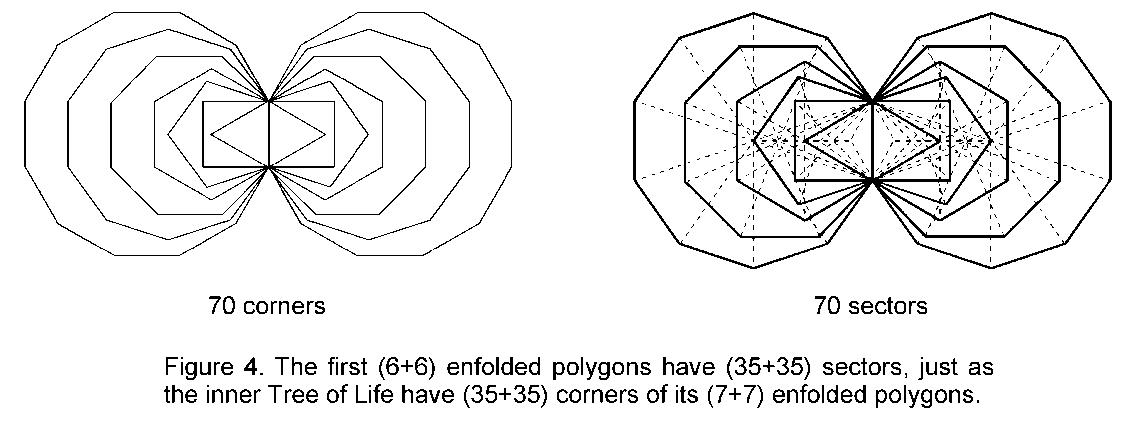4

will confirm that the number 120 is a parameter of holistic systems. It is further evidence that the first (5+5) enfolded polygons constitute such a system.

According to (13), the number of yods on the edges of the 50 tetractyses in the (5+5) enfolded polygons is 224. Two of them coincide with the lowest corners of the two hexagons enfolded in the polygons enfolded in the next higher tree. Hence, there are on the boundaries of the (5+5) enfolded polygons 222 yods that are intrinsic to them alone. The (47+47) tetractyses making up the (7+7) enfolded polygons have 444 hexagonal yods on the edges of their tetractyses, illustrating how the Tetrad (4) expresses this property of the inner Tree of Life.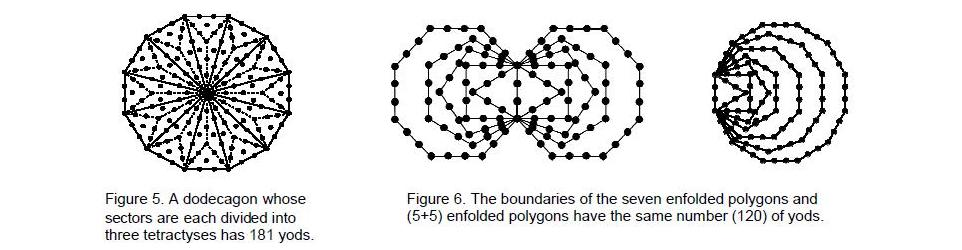222 hexagonal yods are associated with each set of polygons (Fig. 7). The number 222 plays a role in shaping both the seven enfolded polygons and the (5+5) enfolded polygons. It illustrates how the same set of parameters defines different, but equivalent, examples of holistic geometry. We will next discuss some holistic parameters that manifest in physics.

4. Embodiment of some fundamental numbers in physics
Fine-structure number 137
The first (5+5) enfolded polygons have 274 yods (see (11) in the list above). 137 yods are associated with each set of the first five enfolded polygons. Alternatively, a set of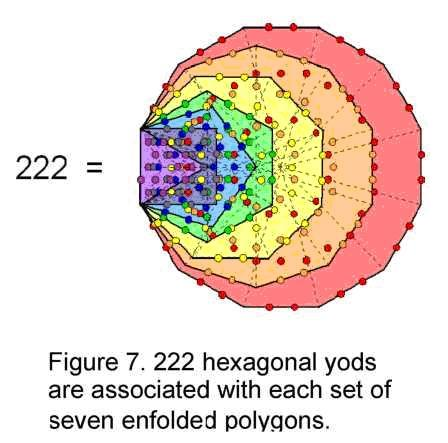five enfolded polygons has 137 yods that are intrinsic to itself in the sense that none of them coincide with yods in polygons enfolded in adjacent trees. The first (5+5) separate polygons have 270 yods on the edges of their 52 tetractyses (see (14) in the list of properties of the (5+5) separate polygons). Hence, if we consider the two sets of these polygons separated by the root edge with four yods in it, there are 274 yods on their boundaries. 137 yods are associated with the separate polygons as well as with the enfolded ones! This is a remarkable feature that is unique to this set. The number 137 is one of the ‘mystery’ numbers in modern physics because its reciprocal is almost equal to the fine-structure constant: e2/ħc ≈ 1/137.035999071, a dimensionless number that measures the strength of the coupling of photons to the electron and which has yet to be derived from a theory that unifies particles and their forces. 137 is the 33rd prime number, where 33 = 1! + 2! + 3! + 4!, i.e., the number of permutations of ten objects arranged in the four rows of a tetractys. Amazingly, the Godname YAHWEH with number value 26 prescribes the very set of polygons with 26 corners whose yod population is the number determining the fine-structure constant . More amazingly still, the number 27, the 26th integer after 1, determines the yod population of the inner Tree of Life because 27 overlapping Trees of Life constructed from tetractyses have 1370 yods .

The first five enfolded polygons have 139 yods, one of which coincides with the lowest corner of the hexagon enfolded in the next higher tree, leaving 138 yods that are intrinsic to either set. The holistic significance of this number is as follows: by including integer powers of 4 as well as the powers of 2 and 3 that make up two sides of the Platonic Lambda Tetractys, it is shown in Article 12  that the latter can be generalised to a tetrahedral array of 20 integers. The sum of the ten powers of 1, 2, 3 & 4 on its edges is:

 138 = 21 22   43 23   20   42   31   32   33   41.

Remarkably, the sum of these primary powers of 1, 2, 3 & 4 is the number of yods intrinsic to the first five

5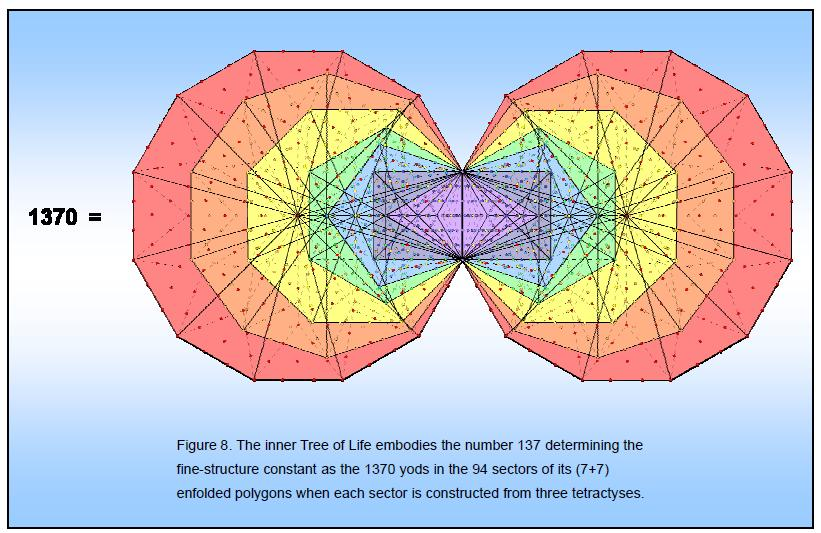6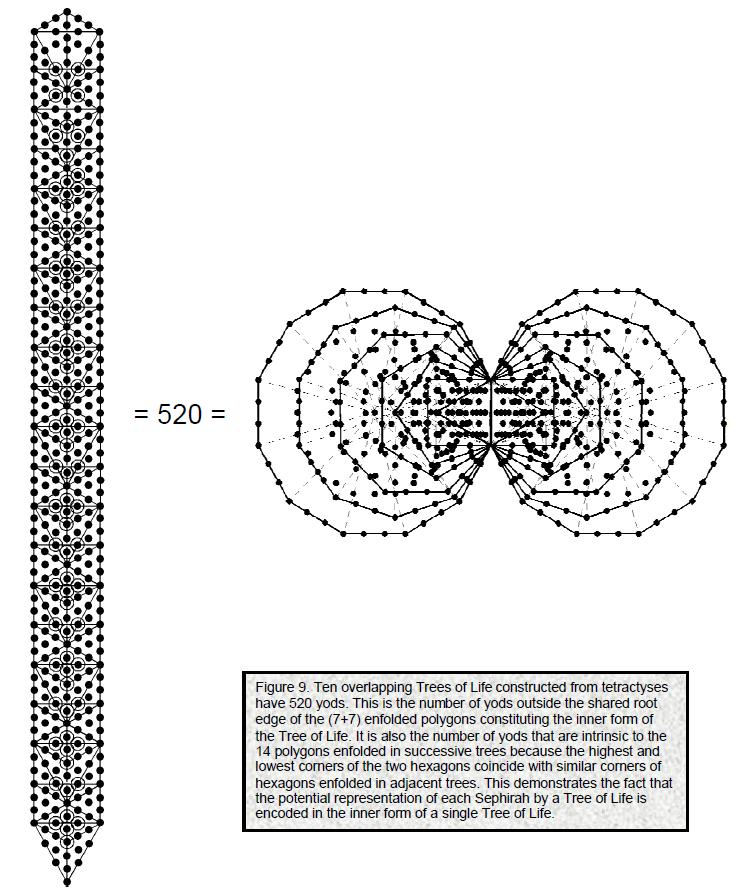enfolded polygons. This demonstrates that the archetypal pattern of integers in the tetrahedral Lambda Tetractys is the arithmetic counterpart of holistic systems possessing sacred geometry. The number 137 determining the fine-structure constant is the sum of the nine powers of 2, 3 & 4 on the edges of this tetractys array. Here is another way in which the tetractys is connected arithmetically to the number 137.

This number manifests in the (7+7) enfolded polygons as their 1370 yods when their sectors are constructed from three tetractyses (Fig. 8). In other words, the yods in 137 tetractyses populate the inner Tree of Life. It is unmistakable evidence that a number of central importance to theoretical physics is

7

embodied in the sacred geometry of the inner Tree of Life.

Dimension 248 of E8

The yod populations of the n-tree and n overlapping Trees of Life are:

 n-treeY(n) = 50n + 30 n Trees of LifeỸ(n) = 50n + 20.

Notice that they are determined by the number value 50 of ELOHIM, Godname of Binah. The 5-tree has (Y(5)= 280) yods, which is the number value of Sandalphon, the Archangel of Malkuth, whilst five overlapping Trees of Life have (Y(5)=270) yods. This is the same as the number of yods outside the root edge of the (5+5) enfolded polygons when their sectors are tetractyses (see (11) in the list of properties of the (5+5) polygons) . Alternatively, it is the number of yods in each set of (5+5) polygons enfolded in successive trees that are intrinsic to them in the sense that none of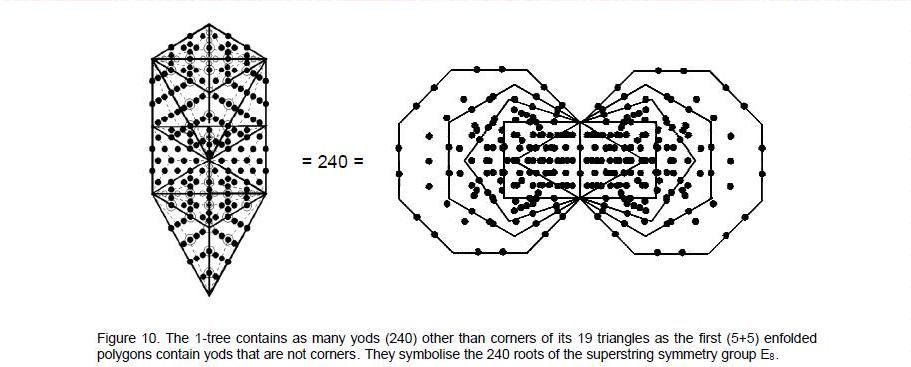their 270 yodsis shared with polygons enfolded in adjacent trees. This is because four yods — the top and bottom corners of the two hexagons enfolded in any tree coincide with, respectively, the bottom and top corners ofhexagons enfolded in adjacent trees, so that, of the 274 yods in each set of (5+5) enfolded polygons, 270 yods are unshared. Ten overlapping Trees of Life have (Ỹ(10)=520) yods. This is the number of yods in the (7+7) enfolded polygons outside their root edge (Fig. 9). Alternatively, it is the number of intrinsic yods in the (7+7) polygons enfolded in every overlapping tree. The two sets of seven enfolded, regular polygons therefore encode ten overlapping Trees of Life — what is generated when each Sephirah of the Tree of Life is represented by another Tree of Life, whilst the first (5+5) enfolded polygons encode five overlapping trees. The Sephiroth are divided in Kabbalah into the uppermost five — Kether, Chokmah, Binah, Chesed & Geburah, which span its Upper Face , and the lowest five — Tiphareth, Netzach, Hod, Yesod & Malkuth, which span its Lower Face. The first (5+5) enfolded polygons are the counterpart of five overlapping trees, whilst all (7+7) polygons are the counterpart of ten trees. More precisely, we can say that the 270 yods intrinsic to the first (5+5) polygons enfolded in every tree correspond to the 270 yods in the uppermost five trees and the remaining 250 yods correspond to the 250 yods in the remainder of the ten trees. The 5:7 division of polygons therefore corresponds to the division of the Tree of Life into its Upper and Lower Faces. The first five polygons define a holistic structure in themselves because they are the inner Tree of Life counterpart of the Upper Face of its outer form. The division of the outer Tree of Life into two halves and the counterpart of this in its inner form have a profound scientific significance, as now explained: 34 of the 274 yods in the (5+5) polygons are corners of polygons, leaving 240 yods (Fig. 10). They symbolize the 240 non-zero roots of E8. Its eight simple roots are symbolised by the yods at the eight corners that coincide either with the three Sephiroth on each side pillar or with the projections of Tiphareth and Daath onto the plane of the polygons. YAHWEH prescribes this representation of the 248 roots of E8 because the (5+5) enfolded polygons have 26 corners other than ones shared with triangles of the outer Tree of Life. It is readily confirmed that 31 of the 248 yods coincide with the projections of the 70 yods of the Tree of Life onto the plane containing the polygons. EL, the Godname of Chesed next below Chokmah on the Pillar of Mercy, prescribes how many yods in the (5+5) enfolded polygons are shared with yods in the outer Tree of Life or

8

their projections.

The 240 yods of the (5+5) enfolded polygons that are not corners have their counterpart in the 1-tree. When its 19 triangles are divided into their sectors and each sector turned into a tetractys, the 1-tree has 251 yods. Their meaning vis-a-vis the E8×E8 heterotic superstring and the mathematical structure of the Cosmic Tree of Life mapping all levels of reality was discussed in Article 5 . Eleven of them are SLs, leaving 240 yods that are generated by the transformation. The 34 corners of the polygons are the counterpart of the 11 corners of the 19 triangles. This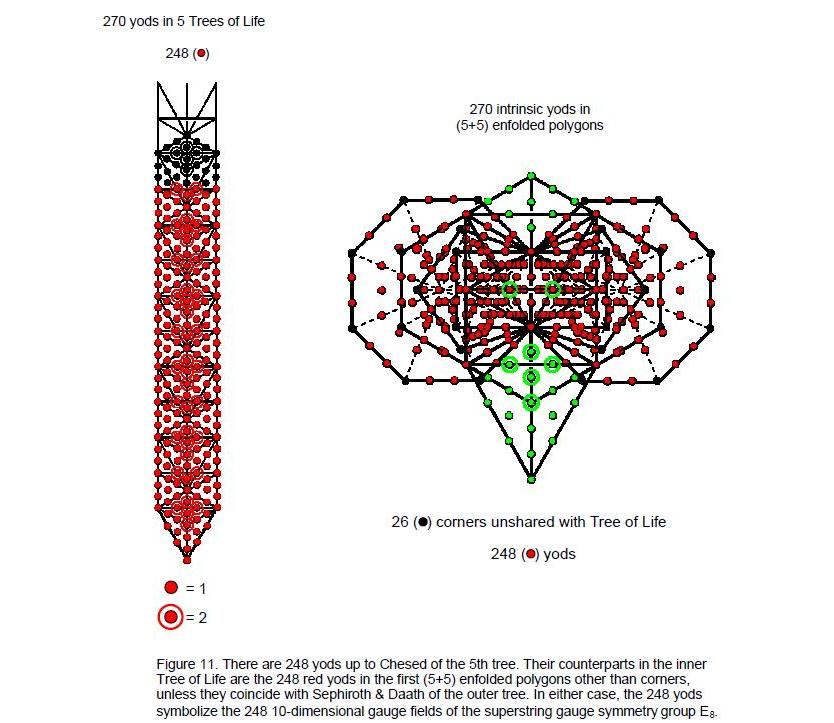embodiment of the number of non-zero roots of E8 appears in many other examples of sacred geometry to be discussed in future articles. It manifests in the five Platonic solids  whose faces are constructed from tetractyses as the 240 hexagonal yods in the 18 faces of the tetrahedron, octahedron & cube, as the 240 hexagonal yods in the 20 faces of the icosahedron and as the 240 hexagonal yods in the 12 faces of the dodecahedron. The average number of yods in the faces of the first four regular polyhedra is 137 . As we saw earlier, this is the number of yods intrinsic to a set of five enfolded polygons. It is more evidence for the archetypal nature of the number 137.

The counterparts in the 5-tree of the 248 yods in the (5+5) enfolded polygons other than their corners are the 248 yods up the 31st SL, Chesed of the 5th tree (Fig. 11). The same 31:217 division appears because the two structures are equivalent and must therefore manifest analogous divisions. The 31 SLs are the counterpart of the 31 yods in the (5+5) enfolded polygons that coincide with yods in the outer Tree of Life when it is projected onto the plane containing them. Sandalphon, the Archangel of Malkuth with number

9

value 280, determines the 5-tree with 280 yods and Raziel, the Archangel of Chokmah with number value 248, determines that section of it containing 248 yods. Tzadkiel, the Archangel of Chesed with number value 62, determines the part of the outer tree that has 62 yods whose projections onto the plane of the polygons do not coincide with any of their corners.

The 1-tree have Y(1) = 80 yods, where 80 is the number value of Yesod (Fig. 12). Above the 1-tree are 168 yods up to the 31st SL, where 168 is the number value of Cholem Yesodeth, the Mundane Chakra of Malkuth. As many subsequent articles will prove, this 80:168 division is characteristic of holistic systems displaying sacred geometry. Its counterpart in the first (5+5) enfolded polygons are the 80 yods in the triangles and hexagons other than corners of the latter that surround their centres and the 168 yods in the other polygons apart from their corners. The 168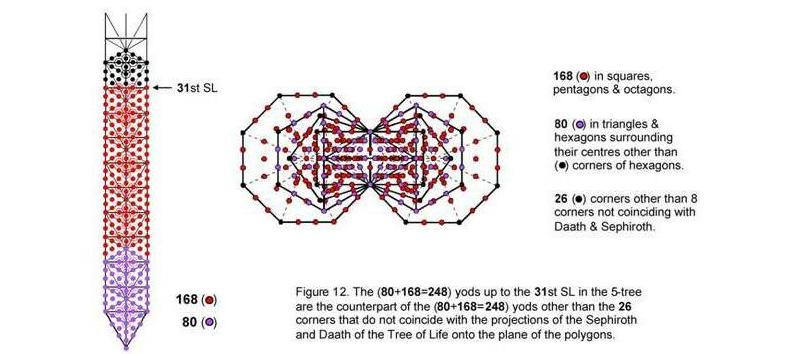yods comprise 84 yods associated with each set of polygons. This 84:84 division is also characteristic of holistic systems, as later articles will demonstrate.

The numbers 168 and 84 are both consistent with the Tetrad Principle because: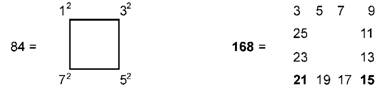The Tetrad also defines the number 248 because there are 248 yods other than corners of tetractyses in a square whose four triangular sectors are so-called '2nd-order tetractyses' (Fig. 13). The tetractys is but the first member ('1st-order tetractys') of an infinite set of tetractyses where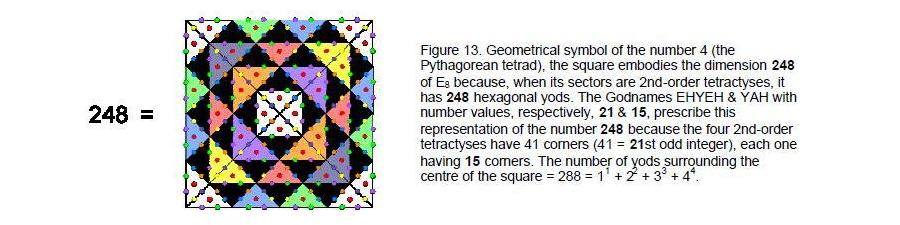successive members are derived

10

from the previous one by turning their yods into 1st-order tetractyses: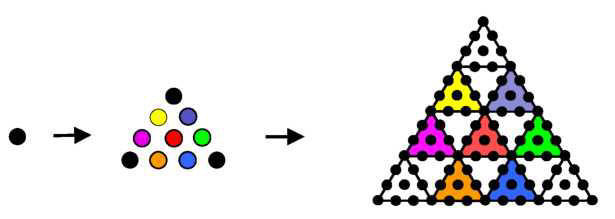The next member — the 2nd-order tetractys — has 85 yods, where

85 = 40 + 41 + 42 + 43,

and 72 yods per sector of a square. Of the latter, 62 are hexagonal yods (see Figure 13), so that the square contains (4×62=248) hexagonal yods. An ancient symbol of the four elements of Earth, Water, Air and Fire, the square actually embodies the number of quantum states of the messenger particle transmitting the unified force between superstrings — the force which shapes the very matter of the universe and determines its properties! Such is the latent power of the Tetrad and its simplest geometrical representation.

The two octagons have 78 yods other than corners. The centres of the triangles, the squares & pentagons number 90 yods other than corners. Amazingly, this reproduces the number values of the two Hebrew words Cholem and Yesodeth: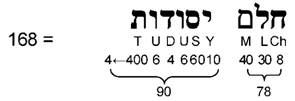It demonstrates that there is a geometrical basis for the Hebrew names of the Sephiroth in the four Kabbalistic Worlds. This is because they collectively prescribe the nature of the universal blueprint.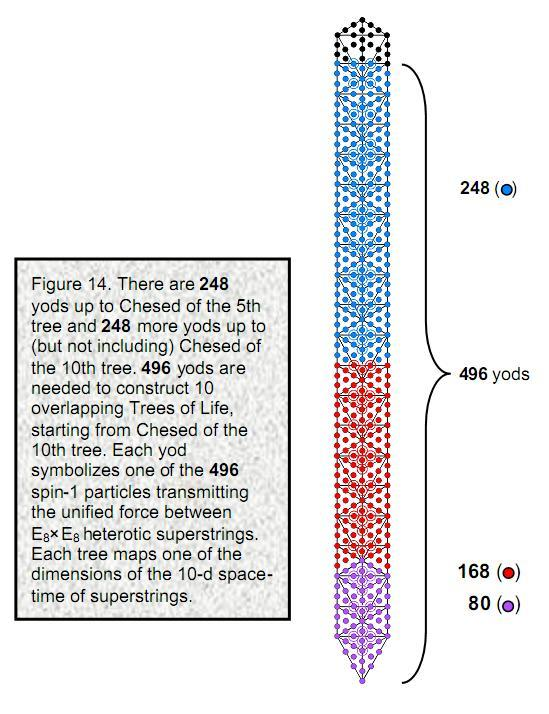The 80 yods other than corners that surround the centres of the triangles and hexagons consist of the two endpoints of the root edge and 78 external yods that comprise six yods coinciding with the Sephiroth on the side pillars and 72 other yods. The 78 yods symbolise the roots of E6, the rank-6 exceptional subgroup of E8, the six yods denoting its six simple roots. The 168 yods symbolise the 168 roots of E8 that are not any of the 72 roots of E6, the two hexagonal yods in the root edge denoting the two simple roots of E8 that are not simple roots of E6. What is being flagged by the division: 248 = 8 + 72 + 168 exhibited by sacred geometries is what physicists call the ‘break-down’ of the symmetry of E8 into the symmetry of E6, which some of them have explored as a possible basis for the Standard Model of particle physics.

Beyond Chesed of the 5th tree, there are 248 more yods up to, but not including, Chesed of the 10th tree, which is the 61st SL (Fig. 14). As we have seen, the uppermost five trees map the Upper Face and the lowest five trees map the Lower Face. The fundamental Kabbalistic distinction between them therefore defines two sets of 248 yods. Fig. 14 shows the 248 blue yods in the five uppermost Trees of Life and the 248 yods in the lowest five Trees of Life, the latter comprising the 80 purple yods in the 1-tree and the 168 red yods above it. 248 is the number value of Raziel, the Archangel of Chokmah, 80 is the number value of Yesod and 168 is the number value of Cholem Yesodeth, the Mundane Chakra of Malkuth.

11

These 496 yods symbolize the 496 roots of the heterotic superstring gauge symmetry group E8×E8. The doubling of E8 is the manifestation of the two Faces of the Tree of Life, which divide its emanation into two sets of five Sephiroth. The other type of heterotic superstring has SO(32) symmetry. It would exist in a universe where there is no distinction between the Upper and Lower Faces of the Tree of Life blueprint governing matter. Given that superstring theory predicts that 496 particles mediate the unified interaction between 10-dimensional superstrings, it would be highly implausible to dismiss as coincidence the fact that 496 yods are needed to generate the emanation of ten Trees of Life, starting from Chesed, the very first Sephirah of Construction, of the tenth tree. EL, its Godname with number value 31, prescribes the number 496 as well as the number 248 because the starting point is the 61st SL, where 61 is the 31st odd integer.

According to (11) in the list of properties of the (5+5) enfolded polygons, their 50 sectors have 133 corners & edges. This is the dimension 133 of E7, the largest exceptional subgroup of E8. According to (8) in the list of properties for the five separate polygons, they have 78 sides and sectors. This is the dimension 78 of E6, another exceptional subgroup of E8. However, because E6 is a subgroup of E7, we should rather expect a subset of the (5+5) enfolded polygons with 78 corners & edges to constitute a proper embodiment. Does one exist? Indeed, it does. Here are the numbers of corners & edges in the root edge and in the (5+5) enfolded polygons outside it ("1" denotes either Daath or a Sephirothic corner):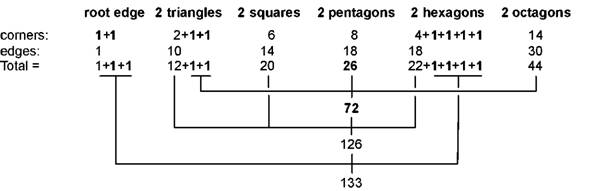The two corners & edge of the root edge and the four Sephirothic corners of the pair of hexagons correspond to the seven simple roots of E7; the remaining 126 corners & edges correspond to its 126 roots. The 72 corners & edges of the pentagon, octagon & Sephirothic corners of the pair of triangles correspond to the 72 roots of E6. The six Sephirothic corners of the root edge and the pair of hexagons correspond to its six simple roots. The (5+5) enfolded polygons are the geometrical counterpart of the root composition of E7 and E6.

As the topmost corners of the two hexagons coincide with the lowest corners of the hexagons enfolded in the next higher tree, there are (133–2=131) corners & edges of the 50 sectors that are intrinsic to them. This is the number value of Samael, the Archangel of Geburah. There are (248–2=246). yods other than non-Sephirothic corners. 246 is the number value of Gabriel, the Archangel of Yesod. Of the 274 yods in the (5+5) enfolded polygons, (274–2=272). yods are intrinsic to them. 272 is the number value of Cherubim, the Order of Angels assigned to Yesod. 248 is the number value of Raziel, the Archangel of Chokmah.

5. Embodiment of the human skeleton
According to (13) in the list of properties of the (5+5) enfolded polygons, there are 224 yods on the boundaries of their 50 tetractyses. They include 34 corners of polygons and 190 hexagonal yods & centres of polygons. The numbers of the latter outside the root edge for each set of polygons are:

 triangle square pentagon hexagon octagon 11 15 19 18 31

Consider the yods of the Tree of life projected onto the plane of the polygons. The centre of the triangle then coincides with a hexagonal yod on the Path connecting Chesed and Geburah.Two hexagonal yods on its lower edge are hexagonal yods on the Path connecting Chesed and Tiphareth, two hexagonal yods on an edge of the hexagon are hexagonal yods on the Path connecting Tiphareth and Netzach and four hexagonal yods on internal edges of tetractyses in the hexagon are hexagonal yods on the Paths connecting Chesed to Chokmah and Netzach. The numbers of yods for each set of polygons unshared with the projected Tree of Life are: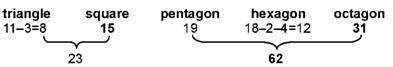12

Including the pair of hexagonal yods in the root edge, on the boundaries of the 50 tetractyses in the (5+5) enfolded polygons there are 34 corners, 23 pairs of unshared yods in the triangles & squares and 63 pairs of unshared yods in the pentagons, hexagons & octagons, that is, 206 yods that are unshared with the projected Tree of Life other than its Sephiroth. Compare this with the fact that the adult human skeleton possesses 206 bones (Fig. 15), of which 34 single bones and 23 pairs of bones constitute the axial skeleton and 63 pairs of bones constitute the appendicular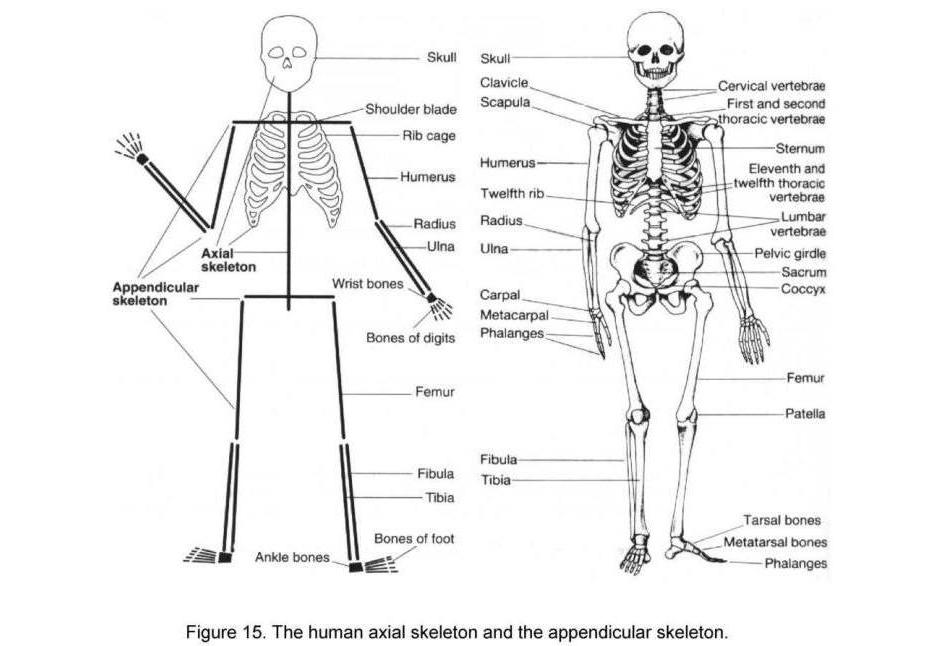skeleton. The 34 corners of the (5+5) enfolded polygons denote the 34 single bones of the core of the skeleton, the 23 pairs of unshared yods on edges of the 14 tetractyses in the pairs of triangles & squares denote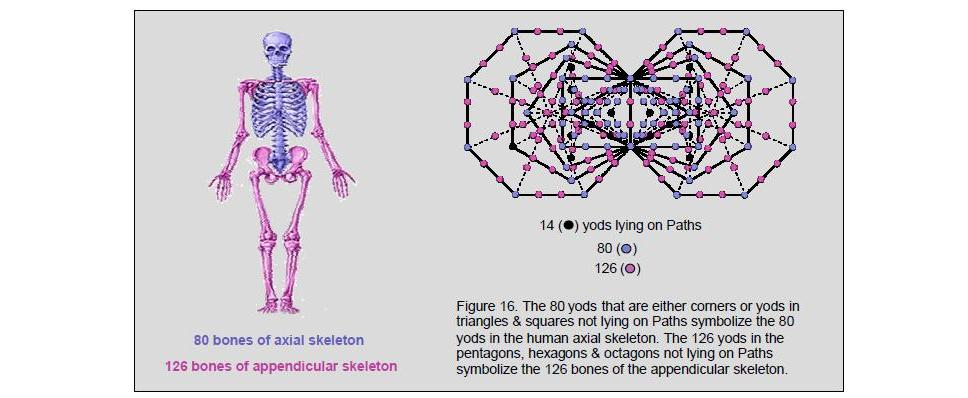13

the 23 pairs of bones in the axial skeleton and the 63 pairs of yods on edges of the 36 tetractyses in the pairs of pentagons, hexagons & octagons denote the 63 pairs of bones in the appendicular skeleton (Fig. 16). The way in which the seven enfolded polygons also embody the 206 bones of the human skeleton will be discussed in Articles 32 & 33 [13, 14].

The 34 yods in a pair of joined triangles are the counterpart of the 34 corners of the first (5+5) enfolded polygons (Fig. 17a). They symbolise the 34 single bones of the axial skeleton. The 23 pairs of yods in two joined squares (Fig. 17b) symbolise the 23 pairs of bones in this skeleton. When the sectors of a triangle and a square are constructed from three tetractyses, a pair of such triangles (Fig. 17c) and a pair of such squares (Fig. 17d) contain 206 yods — the number of bones in the human skeleton. This is a remarkable demonstration of the way in which the tetractys — the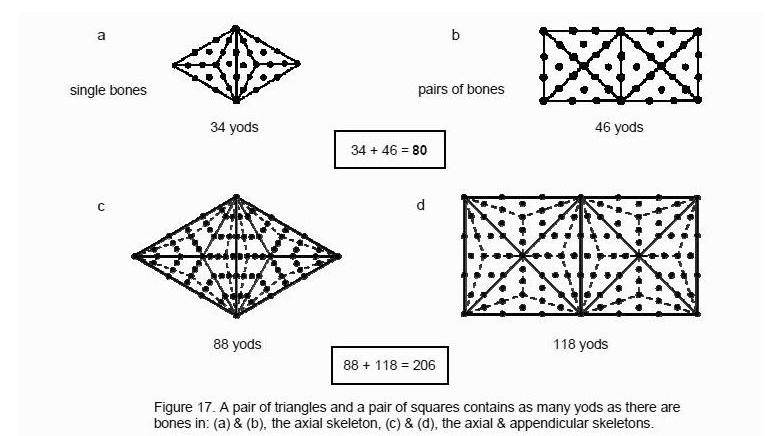template of sacred geometry — generates numbers that parameterise holistic structures like the human skeleton, the Malkuth aspect of the human Tree of Life. Separately, the two types of transformation of the triangle have (19+46=65) yods, where 65 is the number value of ADONAI, the Godname of Malkuth. Separately, the two types of square have (25+61=86) yods. This is the number of pairs of bones in the human skeleton. Joined together, the two types of triangles & squares have 139 yods (Fig 18)  . This is the number of yods in the first five enfolded polygons (see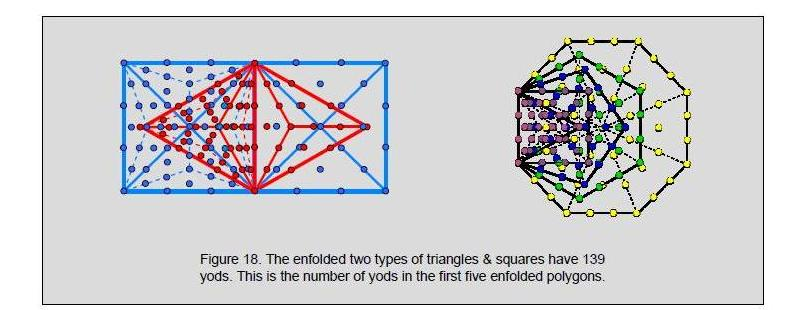(11) in the list of properties of the five enfolded polygons). They are an example of how different holistic systems display the same parameters.

14

6. Conclusion
This article has extended the analysis in previous articles of various subsets of the seven and (7+7) enfolded, regular polygons that constitute the inner form of the Tree of Life. Properties of the new subset — the first five enfolded polygons and the first (5+5) enfolded polygons — were shown to be prescribed by the number values of the ten Hebrew Godnames obtained by gematria. In confirmation of their status as a Tree of Life pattern that embody numbers of cosmic significance, these polygons were shown to contain:

1. 137 yods per set. They therefore embody the number defining the fine-structure constant. As confirmation of their holistic character, the (7+7) enfolded polygons have 1370 yods when their 94 sectors are each constructed from three tetractyses. Also, the tetrahedral Lambda Tetractys has nine powers of 2, 3 & 4 on its edges that sum to 137, the sum of the powers of 1, 2, 3 & 4 being the number of intrinsic yods in a set of the first five enfolded polygons;

2. 240 yods other than their corners. This is the number of yods in the 1-tree other than corners of triangles when the sectors of the latter are constructed from tetractyses. They symbolise the 240 roots of the symmetry group E8, the gauge symmetry group of the unified force between superstrings, the eight corners shared with the outer Tree of Life denoting its eight simple roots. Their counterparts in the 5-tree are the 248 yods up to Chesed of the highest tree. Both exhibit the 80:168 division characteristic of holistic systems (as future articles will demonstrate), whose interpretation in terms of E8 is the difference between the 168 roots of E8 (other than its eight simple roots) that do not belong to its exceptional subgroup E6. The superstring structural parameter 168, which is the number value of Cholem Yesodeth, the Mundane Chakra of Malkuth, is the number of yods in the pairs of squares, pentagons & octagons other than their corners. The number value 78 of Cholem is the number of yods outside the shared edge of the pair of octagons other than their corners. The number value 90 of Yesodeth is the number of yods in the pairs of squares & pentagons other than their corners, together with the centres of the two triangles.
3. 206 yods on the sides of their 50 tetractyses other than those shared with yods on Paths of the Tree of Life. This is the numbers of bones in the human axial and appendicular skeletons. The 34 corners of the (5+5) enfolded corners correspond to the 34 single bones of the axial skeleton, the 23 pairs of yods on edges of tetractyses in the triangles & squares that do not coincide with yods on Paths of the outer Tree of Life when they are projected onto the plane of the polygons denote the 34 pairs of bones in the axial skeleton, and the 63 pairs of unshared yods on edges of tetractyses in the pentagons, hexagons & octagons denote the 63 pairs of bones in the appendicular skeleton. It is remarkable that the number of new yods defining the shapes of the 50 sectors of the (5+5) enfolded polygons, that is, yods that do not lie on Paths of the Tree of Life (apart from the Sephiroth), is the number of bones in the human skeleton. The fact that different groups of yods — most noticeably, the corners — correspond to the numbers of bones making up the two skeletons either as single bones or as pairs strongly argues against the possibility of coincidence. Instead, it is evidence that the bone structure of the human body conforms to the sacred geometry of the Tree of Life. References 12 & 13 analyse in more depth its representation in the outer and inner Trees of Life. The yod populations of the triangle and square reproduce the number of single bones and the number of paired bones in the axial skeleton. Their next level of transformation reproduces the number of bones in the complete skeleton, whilst, enfolded, these polygons have the same yod population as the first five enfolded polygons.

Coincidence also cannot plausibly explain the presence in the same geometrical object of four numbers (137, 240, 248 & 168) that have been shown in previous articles to be connected with either atomic physics or the dynamics and structure of E8×E8 heterotic superstrings, as well as to quantify other Tree of Life patterns independently defined by Godname numbers. The repetition of such fundamental parameters of holistic structures must be understood to represent, instead, different levels of encoding in the Tree of Life of the same basic information about its microphysical manifestation — the superstring.

References

1. Phillips,  Stephen M.  Article  4:  "Godnames prescribe the inner Tree of Life," (WEB, PDF).
2. "The Mathematical Connection between Religion and Science," Stephen M. Phillips (Antony Rowe Publishing, England, 2009).
3. Ref. 1, p. 4.
4. Phillips, Stephen M. Article 3: "The  sacred geometry of the Platonic solids," (WEB, PDF), p. 2.
5. Phillips, Stephen M. Article 11: "Plato's Lambda — its meaning, generalisation and connection to the Tree of Life," (WEB, PDF), p. 5.

15

1. Ref. 1, p. 4.

2. 26 is the number of combinations of ten objects arranged in the four rows ofa tetractys: 1 + 3 + 7 + 15 = 26.
3. Proof: the number of yods in n overlapping Trees of Life ≡ Ỹ(n) = 50n + 20. Therefore, (27) = 1370.
4. Phillips, Stephen M. Article 12: "New Pythagorean aspects of music and their connection to superstrings," (WEB, PDF), p. 2.
5. Phillips, Stephen M. Article 5: "The superstring as macrocosm of the spiritual macrocosm," (WEB, PDF).
6. Ref. 4, p. 11.
7. Ibid, p. 8.
8. Phillips, Stephen M. Article 32: "Derivation of the bone & classical acupoint composition of the human body and their relationship to the seven musical scales," (WEB, PDF).
9. Phillips, Stephen M. Article 33: "The human axial skeleton is the trunk of the Tree of Life," (WEB, PDF).
10. Proof: The numbers of yods in the two types of triangles & squares are:
 triangle square 1st type: 19 25 2nd type: 46 61

There are four yods on each side. Enfolded, the total number of yods = 19 + (25 – 4) + (46 – 4) + (61 – 4) = 139.

16

Home